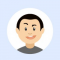## Maximum Erasure Value in C++Kiran P

Updated on 04-Feb-2021 12:01:30

Given an array of positive integers, the task is to erase a subarray containing all the unique elements. What you get by erasing the subarray is equal to the sum of its elements.Return the maximum sum of the current subarray by erasing the terms before or after it, we can ... Read More

## Write a Golang program to find odd and even numbers using bit operationKiran P

Updated on 04-Feb-2021 11:31:15

ExamplesInput num = 5 => 101 & 1 = 001 => True, i.e., Odd; else num would be Even.Approach to solve this problemStep 1: Define a method that accepts a number.Step 2: Perform & operation with that number.Step 3: If the & operator returns a non-zero value, then that number would ... Read More

## Write a Golang program to sort a binary array in linear timeKiran P

Updated on 04-Feb-2021 11:30:28

There are two methods in which we can solve this problem. Let’s check the first method.Method 1ExamplesInput Array = [1, 0, 1, 0, 1, 0, 0, 1] => [0, 0, 0, 0, 1, 1, 1, 1]Approach to solve this problemStep 1: Define a method that accepts an array.Step 2: Count number of ... Read More

## Write a Golang program to find duplicate elements in a given arrayKiran P

Updated on 04-Feb-2021 11:30:03

ExamplesInput Array = [1, 3, 5, 6, 1] => Duplicate element is 1;Input Array = [1, 3, 5, 6, 7] => return -1Approach to solve this problemStep 1: Define a method that accepts an array.Step 2: Declare a visited map.Step 3: Iterate the given array. If the element exists in the visited ... Read More

## Write a Golang program to find duplicate elements in a given rangeKiran P

Updated on 04-Feb-2021 11:29:49

We can solve this problem in two different ways. Let’s check the first method.Method 1: ExamplesInput Array = [1, 2, 3, 4, 4] => Range is from 1 to 5 but 4 is a duplicate element in this range.Approach to solve this problemStep 1: Define a method that accepts an array.Step 2: Declare ... Read More

## Write a Golang program to find pairs with the given sum in an array(O(n))Kiran P

Updated on 04-Feb-2021 11:23:55

ExamplesInput Array = [1, 3, 5, 7, 8, 9], sum = 11 => (3, 8)Approach to solve this problemStep 1: Define a method that accepts an array and sum.Step 2: Define a mapping variable, type map[int]int.Step 3: Iterate the given array as i.Step 4: If key in mapping sum-arr[i] is not present, ... Read More

## Write a Golang program to find pairs with given sum in an array(O(nlogn))Kiran P

Updated on 04-Feb-2021 11:23:38

ExamplesInput Array = [1, 3, 5, 7, 8, 9], sum = 11 => (3, 8)Approach to solve this problemStep 1: Define a method that accepts an array and sum.Step 2: Sort the given array, declare low:=0 and high:=size-1 variables.Step 3: Iterate a for loop until low sum, then high--.Step 5: At ... Read More

## Write a Golang program to find pairs with the given sum in an array(O(n2))Kiran P

Updated on 04-Feb-2021 11:23:24

ExamplesInput Array = [4, 1, 6, 8, 7, 2, 3], sum = 11 => (4, 7) or (8, 3)Approach to solve this problemStep 1: Define a method that accepts an array and sum.Step 2: Iterate from 0 to n as i.Step 3: Again, iterate a for loop from i+1 to n-1 as ... Read More

## Write a Golang program to find the frequency of each element in an arrayKiran P

Updated on 04-Feb-2021 11:15:26

ExamplesInput Array = [1, 3, 4, 3, 2, 3, 4, 0, 2]Element13420Frequency13221Approach to solve this problemStep 1: Define a method that accepts an array.Step 2: Define a map, where key would be the array’s elements and the starting value is 0.Step 3: Start iterating the input array. If an element is present ... Read More

## Write a Golang program to check whether a given array is sorted or not (Using Bubble Sort Technique)Kiran P

Updated on 04-Feb-2021 11:15:04

ExamplesInput arr = [7, 15, 21, 26, 33] => Array is already sorted.Input arr = [7, 5, 1, 6, 3] => Array is not sorted.Approach to solve this problemStep 1: Iterate the array from the 0th index to n-1.Step 2: Iterate the array from the 0th index to n-1-i, where ... Read More

1 2 3 4 5 ... 13 Next